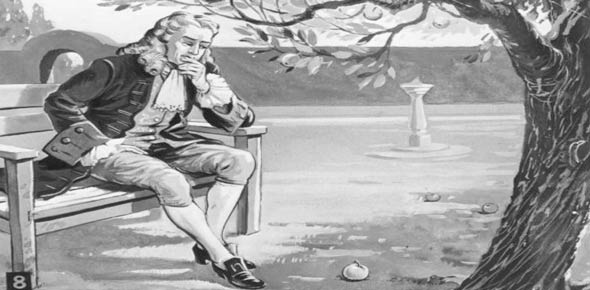# Newton's First Law Review

8 Questions | Attempts: 923
ShareSettingsA review of the basic concepts associated with Newton's First law such as Inertia,net Force and equilibrium

• 1.
Newton's First law is also known as the law of...
• A.

Movement

• B.

Complication

• C.

Inertia

• D.

Inability

• E.

Equal and oopposite forces

• 2.
An object in motion will _____________________ if the Net force acting on it is____
• A.

Stay in motion, 0 N

• B.

Accelerate, 0 N

• C.

Stay in motion,greater than 0 N

• 3.
If an object is in equilibrium its acceleration is _________ and its Net force is _____
• A.

Non-zero, non-zero

• B.

Zero,non-zero

• C.

Zero, zero

• D.

Non-zero,zero

• 4.
Johnny pushes with a force of 50 N to the left, Jill pushes on the car with  a force of 75 N to the right. What is the net force acting on the object?
• A.

125 N

• B.

100 N

• C.

50 N

• D.

25 N

• E.

0 N

• 5.
Johnny pushes with a force of 50 N to the left, Jill pushes on the car with  a force of 75 N to the right. Is the object in equilibrium?
• A.

Yes

• B.

No

• C.

Maybe

• 6.
An object is moving at a constant velocity. If the Car requires 1000 N of force to propel it forward, What is the magnitue of the frictional force acting on the car?
• A.

0 N

• B.

500 N

• C.

1000 N

• D.

1500N

• E.

1000 N

• 7.
An object is moving at a constant velocity. If the Car requires 1000 N of force to propel it forward, Is the car in equilibrium?
• A.

Yes

• B.

No

• C.

Maybe

• 8.
What is the approximate weight of a 10 kg dog?
• A.

1 N

• B.

10 N

• C.

100N

• D.

1000 N

## Related TopicsBack to top
×

Wait!
Here's an interesting quiz for you.